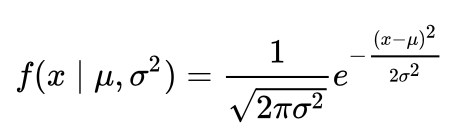# What is a Probability Distribution?

In an experiment, it is universal that each possible value of the random variable has a specific probability of happening.

If you perform an experiment and draw many random samples, the resulting experiment values against their probability of happening are your probability distribution.

You can obtain the probability of each value happening by weighting its frequency during the experiment. A very important point here is that the outcomes of your experiment will most likely be obtained by some measurement such as temperature or by chance such a rolling a dice.

Below Fig 1 and Fig 2 illustrate the probability distribution of the number of orders received by a company per week in the form of a table and a histogram.

# The Normal Distribution and its PDF

The Normal Distribution is a continuous probability distribution that is described by the Probability Density Function (PDF).

The PDF describes the probability of a certain value of the experiment that lies within a particular range of values. It includes a normalizing constant that ensures the area under the curve is equal to one.

The area is equal to 1 because the sum of all events in probability equals 1.This is the probability density function of Normal distribution.

The shape of the Normal Distribution curve is based on the mean and standard deviation of the sample; the curve will be centered and symmetric around the mean and stretched by the standard deviation.

The PDF curve never crosses or touches the x-axis; therefore, it is non-zero across the entire real line. This means, the normal distribution can give you the probability of any event happening, but as it gets farther from the mean, its probability of happening will be closer and closer to zero.

The Empirical Rule (68–95–99.7% rule) states that, in a normal distribution, almost all data lies within 3 standard deviations of the mean. This comes very handily when you are trying to identify outliers in your data or even as a way to check the distribution’s normality.

# How can you determine if your Probability Distribution is Normal?

## Histogram

When you get the sample of outcomes from our experiment, a common first step is to plot the number of occurrences against sample values to get the distribution curve.

When working with Normal Distribution, you should get a bell-shaped curve. If you see a rough estimation of a bell, you can proceed with other tests to be fully sure that your samples come from a normal distribution.

# Q-Q plot

Q-Q plot helps you determine whether your dependent variable comes from a normal distribution or not. Q-Q plots take theoretical normal distribution quantiles which is our x-axis and compare them against your sample data quantiles which are our y-axis. If both sets come from a normal distribution, then the scatter plot will roughly form a straight line with a 45-degree angle.

Just like the histogram, the Q-Q plot is a visual check and it is subjective to what the reader might consider a good-enough straight line is.

# Additional Statistical Tests

You can also do some additional tests to confirm the normality of your probability distribution. A common statistical test for normality is the Shapiro-Wilk test, which tells you if your data comes from a normal distribution depending on the alpha level you have set.

# REFERENCES

Don’t forget to leave your responses.

Everyone stay tuned!! To get my stories in your mailbox kindly subscribe to my newsletter.

Thank you for reading! Do not forget to give your claps and to share your responses and share it with a friend.

--

--